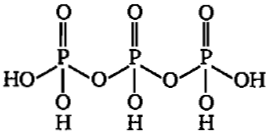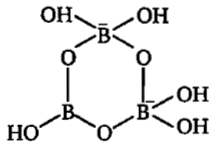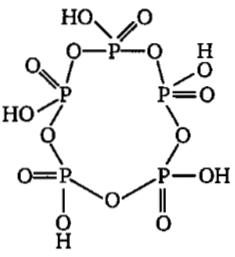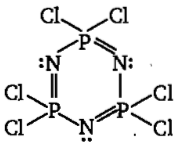# NEET Chemistry The p-Block Elements (XII) Questions Solved

Among the following cyclic species are :

(c)$\left(\mathrm{I}\right)$                                                       $\left(\mathrm{II}\right)$$\left(\mathrm{III}\right)$                                                $\left(\mathrm{IV}\right)$

Difficulty Level:

• 7%
• 37%
• 35%
• 24%